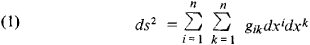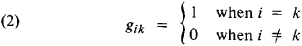# Metric Tensor

Also found in: Wikipedia.

## metric tensor

[′me·trik ′ten·sər]
(mathematics)
A second rank tensor of a Riemannian space whose components are functions which help define magnitude and direction of vectors about a point. Also known as fundamental tensor.
McGraw-Hill Dictionary of Scientific & Technical Terms, 6E, Copyright © 2003 by The McGraw-Hill Companies, Inc.
The following article is from The Great Soviet Encyclopedia (1979). It might be outdated or ideologically biased.

## Metric Tensor

the set of quantities that define the geometric properties of a space (the metric of the space). In the general case of an n -dimensional Riemannian space, the metric is defined as the square of the distance ds2Pointe (x1,x2,.......,xn) and (x1 + dx1, x2 + dx2,...., xn)+ dxn)where xl, x2, . . . , xn are coordinates and the gik are certain functions of the coordinates. The set of the quantities gik forms a second-rank tensor, which is called the metric tensor. This tensor is symmetric, that is, gik = gki The form of the components of the metric tensor gik depends on the choice of the coordinate system, but ds2 does not change in changing from one coordinate system to another, that is, it is invariant with respect to transformations of coordinates. If the metric tensor can be reduced to the formbetween two infinitesimally close by selection of the coordinate system, then the space is a plane, Euclidean space. (For a three-dimensional space, ds2 = dx2 + dy2 + dz2, where x1 = x, x2 = y, and x3 = z are the rectangular Cartesian coordinates.) If a metric tensor cannot be reduced to the form (2) by any transformation of coordinates, then the space is curved and the curvature of the space is defined by the metric tensor. In the theory of relativity, the space-time metric is defined by a metric tensor.

G. A. ZISMAN

References in periodicals archive ?
His topics include basis vectors and scale factors, contravarient components and transformations, metric tensor operation on tensor indices, Cartesian tensor transformation--rotations, and a collection of relations for selected coordinate systems.
where [R.sub.ab] is the Ricci tensor, R is the scalar curvature, [g.sub.ab] is the metric tensor, [LAMBDA] is the cosmological constant, G is Newton's gravitational constant, and c is the speed of light constant.
The torsion free space ([V.sub.4]) is represented by a Riemannian manifold M of four dimensions having signature (+, -, -, -) with metric tensor [g.sub.ab] and symmetric stress-energy tensor [T.sub.ab] (a, b = 0, 1, 2, 3).
where, R, is the Ricci scalar curvature, and gab is the fundamental metric tensor.
Let ([M.sup.n],g) be an n-dimensional Riemannian manifold of class [C.sup.[infinity]] with the metric tensor g and [nabla] be the Riemannian connection of the manifold ([M.sup.n], g).
During the strategy, one of the crucially important rules is to view the nonuniform mesh (i.e., the physical mesh in this paper) as a uniform mesh under the metric tensor M; the tensor M is a symmetric and positive definite matrix.
In 1925, Levy  proved that "A second order covariant constant nonsingular symmetric tensor in a space of constant curvature is proportional to the metric tensor." Thus, a semi-Riemannian manifold of constant curvature admits no ACV other than a CKV.
Concretely, the metric tensor, the determinant of metric matrix field, the Christoffel symbols, and Riemann tensors on the 3D domain are expressed by those on the 2D surface, which are featured by the asymptotic expressions with respect to the variable in the direction of thickness of the shell.
In this paper, we will first construct two metric tensor fields; through these metric tensor fields, sinh-Gordon equations and elliptic sinh-Gordon equation are obtained.
Transformation rules between base vectors are given by [[bar.e].sub.i] = [g.sup.ij] [[bar.e].sup.j], [[bar.e].sub.i] = [g.sub.ij][[bar.e].sup.j], where [g.sub.ij] = [[bar.e].sub.i] x [[bar.e].sub.j] is the metric tensor.

Site: Follow: Share:
Open / Close